Solving a Puzzle, Mathematically

loopspace

20th April 2016

# 1 Introduction

In A Tale of Two Puzzles, I argued that there is a difference between solving a puzzle and doing mathematics. This is particularly evident when the puzzle has artificial constraints, or is purely a why question1. To make my point clear, I'm going to approach a puzzle mathematically. I'm going to pretend that I've come across this in my research somehow and need to solve it to move on to the next bit.

1Where you're given the answer and asked to explain the "neat" reason why it is so.

# 2 The Puzzle

This is a famous puzzle (my book attributes it to Langley). It's an angle problem, which is supposed to be solvable without using trigonometry. There are a few equivalent ways of specifying the puzzle, and the unknown angle might be a different one (there are four which if you can work out one then the other three follow easily), I'll start with the formulation as in Figure 1.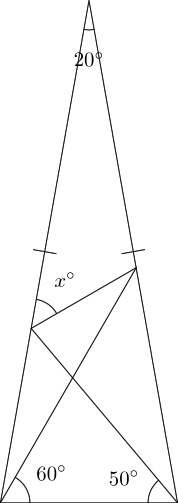Figure 1: Find the angle ${x}^{\circ }$.

You can fill in various angles quite easily, but none of them get you directly to ${x}^{\circ }$.

There is clearly some cunning construction that gives you the angle. However, after having stared at this diagram for some time2 it hasn't occurred to me. So I'm going to "do the math" and just calculate the thing.

2We're talking years here.

Actually, I'm not even going to do that. I'm going to measure it. Or rather, my computer is going to. The program I'm using to draw these diagrams (which, contrary to standard practice, are drawn to scale) can measure them. Figure 2 is an accurate drawing.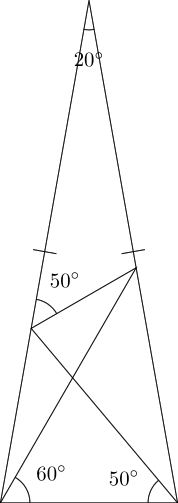Figure 2: Found the angle ${x}^{\circ }$.

So, now I'm done. I've solved the problem. Well, not quite. If this were a genuine problem I'd come across where I didn't know the answer then I wouldn't know for sure if that ${50}^{\circ }$ was exact or just a coincidence of rounding. As it's from a puzzle, I can be confident that the answer is going to be a "nice" answer and so am confident that ${50}^{\circ }$ is correct.

As a mathematician, I'm instantly curious as to the coincidence of the two ${50}^{\circ }$ angles. I want to know if there is a reason for that or if it genuinely is a coincidence.

# 3 Do The Experiment

There's an obvious hypothesis: that the found angle is equal to the right-hand base angle. I must admit that I doubt this one (because it should also depend on the ${60}^{\circ }$ and ${20}^{\circ }$), but it's worth a test. The way to test this is to vary the ${50}^{\circ }$, keeping the other two fixed.

Varying only the ${50}^{\circ }$, we see that ${x}^{\circ }$ is not usually equal to the base angle. We do see some angles where ${x}^{\circ }$ is "nice", such as ${70}^{\circ }$ on the base giving ${x}^{\circ }$ as ${130}^{\circ }$, but there is no obvious pattern. Table 1 gives the list of "nice" pairs. Varying only the ${60}^{\circ }$ gives Table 2. For the top angle, only ${20}^{\circ }$ gives a "nice" angle for ${x}^{\circ }$.

 Base angle ${x}^{\circ }$ ${70}^{\circ }$ ${130}^{\circ }$ ${65}^{\circ }$ ${105}^{\circ }$ ${60}^{\circ }$ ${80}^{\circ }$ ${50}^{\circ }$ ${50}^{\circ }$ ${47}^{\circ }$ ${45}^{\circ }$ ${35}^{\circ }$ ${33}^{\circ }$ ${30}^{\circ }$ ${30}^{\circ }$

Table 1: Right-hand base angles giving "nice" angles for ${x}^{\circ }$

 Base angle ${x}^{\circ }$ ${70}^{\circ }$ ${20}^{\circ }$ ${60}^{\circ }$ ${50}^{\circ }$ ${50}^{\circ }$ ${80}^{\circ }$ ${40}^{\circ }$ ${100}^{\circ }$ ${20}^{\circ }$ ${120}^{\circ }$

Table 2: Left-hand base angles giving "nice" angles for ${x}^{\circ }$

Obviously, one could explore more by varying the three together to find other triples that work. But this does not appear to be a fruitful line of enquiry. It might be illuminating to draw some graphs of ${x}^{\circ }$ against the base angles to spot the connecting function, but there are other avenues to explore first.

# 4 Spot The Coincidences

Let's fill in all the angles, as in Figure 3. There are a lot of angles that agree in that diagram. Although the four difficult angles (the ${50}^{\circ }$, ${100}^{\circ }$, ${80}^{\circ }$, and ${30}^{\circ }$ on that midline) can also be found elsewhere, there are no obvious connections between them.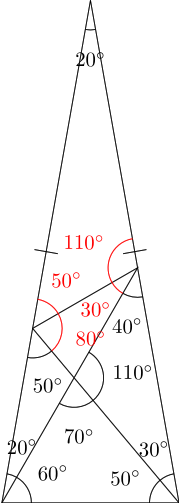Figure 3: All the angles. The red angles are the ones to find.

However, one thing that the overcrowded Figure 3 shows is that there are quite a few isosceles triangles here. There is the outer one, but then also one along the left-hand side with "base" angles ${20}^{\circ }$ and another at the bottom with "base" angles ${50}^{\circ }$. Maybe the key is keeping these triangles isosceles. This gives us only one degree of freedom, which can be chosen to be the angle at the top of the outer triangle. Once this is fixed, the other two are determined by the isosceles requirements.

 Top angle ${x}^{\circ }$ ${9}^{\circ }$ ${13}^{\circ }$ ${20}^{\circ }$ ${50}^{\circ }$ ${36}^{\circ }$ ${108}^{\circ }$

Table 3: Special isosceles triangles

The top angles that give "nice" angles for ${x}^{\circ }$ are given in Table 3. There aren't many! This suggests that the secret is more complicated than that the outer triangle is isosceles.

# 5 Superposition

Another route from one angle to another is to find two parts of the diagram that are similar (in the proper mathematical sense). There are two similar triangles in the diagram. The top triangle and bottom left triangles are both ${20}^{\circ }-{50}^{\circ }-{110}^{\circ }$. There are also two ${20}^{\circ }-{30}^{\circ }-{110}^{\circ }$ triangles.

Both of these use the same ${20}^{\circ }$ angles so let's take our diagram and super-impose it on itself so that the two ${20}^{\circ }$ angles agree. We then have a choice as to how big to make the diagram. We can either align the ${50}^{\circ }$ angles or the ${30}^{\circ }$ angles. The drawing is slightly easier to do if we align the ${30}^{\circ }$ angles so we'll do that. The result is in Figure 4 with the original diagram in black and the overlay in red.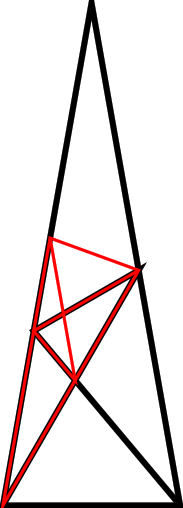Figure 4: The diagram super-imposed on itself.

Well, now, there's a thing. The red version of the diagram is drawn so that the ${20}^{\circ }$ and ${30}^{\circ }$ angles coincide, but it turns out that that ${50}^{\circ }$ angles also coincide.

Looking at this diagram, I find myself wanting to repeat the superposition construction.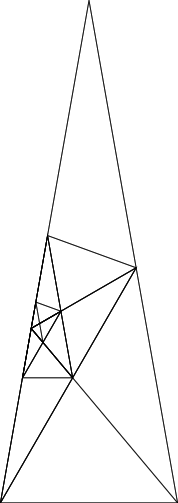Figure 5: The diagram super-imposed on itself repeatedly.

This gives a sequence of triangles that appear to be converging to the point where the cross line meets the left-hand edge of the original triangle.

Let's try to give a concrete description of this process. Start with a non-equilateral triangle and pick two unequal angles. Construct a scaled copy of the triangle inside with the following properties:

1. The vertex with the smaller of the chosen angles in the copy is at the vertex with the larger chosen angle in the original.

2. The edge between the chosen angles on the smaller triangle lies along the edge between the chosen angles on the original.

3. The triangle is as large as it can be.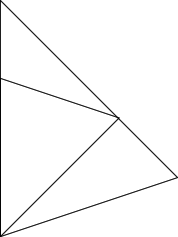Figure 6: The first stage of the construction.

Then we iterate the process to produce Figure 7.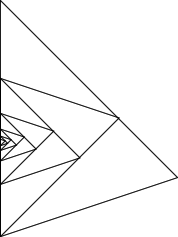Figure 7: After ten iterations.

It's a straightforward argument to show that the third vertices of each triangle form two families lying along two straight lines which intersect at the convergence point3. Moreover, these two lines meet the edge of the original triangle at the same angle (albeit, one from above and one from below).

3I'm strongly resisting the temptation to write "Banach's fixed point theorem" here. Incidentally, did you know that if you place a map of your city on your dining room table then there is a unique point on the map which is in exactly the right place?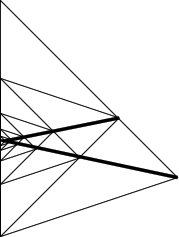Figure 8: With the joining lines.

In order to show that this is truly what is going in our original diagram, we need to show that the lines genuinely match. That is, in Figure 4 we aligned the ${30}^{\circ }$ angles and observed that the ${50}^{\circ }$ angles part way down the side seemed to match as well. We need to show that this apparent match is actual. One way to do that is to look at ratios of side lengths. Let's label a few vertices.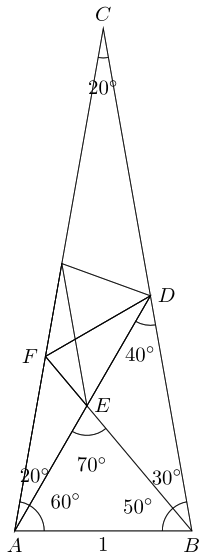Figure 9: The superposition with labelled vertices and angles.

We need to show that the ratio $BD:BC$ is the same as $DE:DA$. It will be slightly easier to work with $EA$ than $DE$ in which case we'll try to show that:

 $\frac{BD}{BC}+\frac{EA}{DA}=1$

We'll use the sine rule to get at the various lengths. As the whole question is about angles, the overall size of the diagram doesn't matter so we're free to set the length of one side. As in Figure 9 we'll set the base to $1$.

The sine rule then says that:

 $\begin{array}{rl}\frac{BC}{\mathrm{sin}\left({80}^{\circ }\right)}& =\frac{1}{\mathrm{sin}\left({20}^{\circ }\right)}\\ \frac{BD}{\mathrm{sin}\left({60}^{\circ }\right)}& =\frac{1}{\mathrm{sin}\left({40}^{\circ }\right)}\\ \frac{DA}{\mathrm{sin}\left({80}^{\circ }\right)}& =\frac{1}{\mathrm{sin}\left({40}^{\circ }\right)}\\ \frac{EA}{\mathrm{sin}\left({50}^{\circ }\right)}& =\frac{1}{\mathrm{sin}\left({70}^{\circ }\right)}\end{array}$

Thus the sum we want to examine is:

 $\begin{array}{rl}\frac{BD}{BC}+\frac{EA}{DA}& =\frac{\mathrm{sin}\left({60}^{\circ }\right)}{\mathrm{sin}\left({40}^{\circ }\right)}\frac{\mathrm{sin}\left({20}^{\circ }\right)}{\mathrm{sin}\left({80}^{\circ }\right)}+\frac{\mathrm{sin}\left({50}^{\circ }\right)}{\mathrm{sin}\left({70}^{\circ }\right)}\frac{\mathrm{sin}\left({40}^{\circ }\right)}{\mathrm{sin}\left({80}^{\circ }\right)}\\ & =\frac{\mathrm{sin}\left({60}^{\circ }\right)\mathrm{sin}\left({20}^{\circ }\right)\mathrm{sin}\left({70}^{\circ }\right)+\mathrm{sin}\left({50}^{\circ }\right)\mathrm{sin}\left({40}^{\circ }\right)\mathrm{sin}\left({40}^{\circ }\right)}{\mathrm{sin}\left({40}^{\circ }\right)\mathrm{sin}\left({70}^{\circ }\right)\mathrm{sin}\left({80}^{\circ }\right)}& & \text{Common denominator}\\ & =\frac{\mathrm{sin}\left({60}^{\circ }\right)\mathrm{sin}\left({20}^{\circ }\right)\mathrm{cos}\left({20}^{\circ }\right)+\mathrm{cos}\left({40}^{\circ }\right)\mathrm{sin}\left({40}^{\circ }\right)\mathrm{sin}\left({40}^{\circ }\right)}{\mathrm{sin}\left({40}^{\circ }\right)\mathrm{sin}\left({70}^{\circ }\right)\mathrm{sin}\left({80}^{\circ }\right)}& & \mathrm{cos}\left(\theta \right)=\mathrm{sin}\left({90}^{\circ }-\theta \right)\\ & =\frac{\mathrm{sin}\left({60}^{\circ }\right)\mathrm{sin}\left({40}^{\circ }\right)+\mathrm{sin}\left({80}^{\circ }\right)\mathrm{sin}\left({40}^{\circ }\right)}{2\mathrm{sin}\left({40}^{\circ }\right)\mathrm{sin}\left({70}^{\circ }\right)\mathrm{sin}\left({80}^{\circ }\right)}& & \mathrm{sin}\left(\theta \right)\mathrm{cos}\left(\theta \right)=\frac{1}{2}\mathrm{sin}\left(2\theta \right)\\ & =\frac{\mathrm{sin}\left({60}^{\circ }\right)+\mathrm{sin}\left({80}^{\circ }\right)}{2\mathrm{sin}\left({70}^{\circ }\right)\mathrm{sin}\left({80}^{\circ }\right)}& & \text{Cancellation}\\ & =\frac{2\mathrm{sin}\left({70}^{\circ }\right)\mathrm{cos}\left({10}^{\circ }\right)}{2\mathrm{sin}\left({70}^{\circ }\right)\mathrm{sin}\left({80}^{\circ }\right)}& & \mathrm{sin}\left(2\theta \right)+\mathrm{sin}\left(2\varphi \right)=2\mathrm{sin}\left(\theta +\varphi \right)\mathrm{cos}\left(\theta -\varphi \right)\\ & =\frac{2\mathrm{sin}\left({70}^{\circ }\right)\mathrm{sin}\left({80}^{\circ }\right)}{2\mathrm{sin}\left({70}^{\circ }\right)\mathrm{sin}\left({10}^{\circ }\right)}& & \mathrm{cos}\left(\theta \right)=\mathrm{sin}\left({90}^{\circ }-\theta \right)\\ & =1\end{array}$

Thus the apparent coincidence is genuine.

This is now enough to prove that the angle ${x}^{\circ }$ is ${50}^{\circ }$. The superposition argument says that ${x}^{\circ }=A\stackrel{^}{F}B$ as the lines $DF$ and $BF$ are the two joining lines from the superposition. Since we know that angle $A\stackrel{^}{F}B={50}^{\circ }$, this shows that ${x}^{\circ }={50}^{\circ }$.

Taking the superposition line then the problem is backwards. In the iteration set-up, one would start with the triangle, apply the construction, and then work out the angle of the new lines which would include the angle going to the third vertex of the original triangle (that's angle $F\stackrel{^}{B}A$ in Figure 4). In the iteration, that angle is not known at the outset. So really one should start knowing that the line $FB$ is from the iteration process and work out angle $F\stackrel{^}{B}A$, rather than knowing angle $F\stackrel{^}{B}A$ and working out that the line $FB$ is from the iteration.

So let's solve this problem in general. We need to generalise our diagram to Figure 10. In this diagram, $\alpha$, $\beta$, and $\gamma$ are specified at the outset as the angles of the triangle. We want to find out angle $\theta$.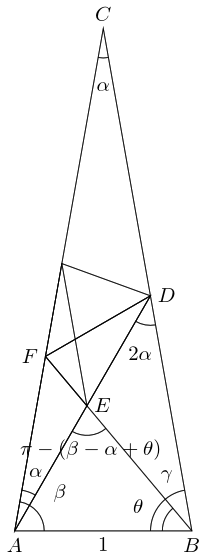Figure 10: The general superposition diagram.

The sine rule then says that:

 $\begin{array}{rl}\frac{BC}{\mathrm{sin}\left(\beta \right)}& =\frac{1}{\mathrm{sin}\left(\alpha \right)}\\ \frac{BD}{\mathrm{sin}\left(\beta -\alpha \right)}& =\frac{1}{\mathrm{sin}\left(2\alpha \right)}\\ \frac{DA}{\mathrm{sin}\left(\gamma \right)}& =\frac{1}{\mathrm{sin}\left(2\alpha \right)}\\ \frac{EA}{\mathrm{sin}\left(\theta \right)}& =\frac{1}{\mathrm{sin}\left(\pi -\left(\beta -\alpha +\theta \right)\right)}=\frac{1}{\mathrm{sin}\left(\beta -\alpha +\theta \right)}\end{array}$

Since we know that this is the line from the iteration, we know that

 $\frac{BD}{BC}+\frac{EA}{DA}=1$

Substituting, we have:

 $\frac{\mathrm{sin}\left(\beta -\alpha \right)}{\mathrm{sin}\left(2\alpha \right)}\frac{\mathrm{sin}\left(\alpha \right)}{\mathrm{sin}\left(\beta \right)}+\frac{\mathrm{sin}\left(\theta \right)}{\mathrm{sin}\left(\beta -\alpha +\theta \right)}\frac{\mathrm{sin}\left(2\alpha \right)}{\mathrm{sin}\left(\gamma \right)}=1$

Using a fair amount of standard trigonometry, we eventually arrive at:

 $\mathrm{tan}\left(\theta \right)=\frac{{\mathrm{sin}}^{2}\left(\gamma \right)\mathrm{sin}\left(\beta -\alpha \right)}{2\mathrm{cos}\left(\alpha \right)\mathrm{sin}\left(2\alpha \right)\mathrm{sin}\left(\beta \right)-{\mathrm{sin}}^{2}\left(\gamma \right)\mathrm{cos}\left(\beta -\alpha \right)}$

As a check, let's put $\beta =\gamma ={80}^{\circ }$ and $\alpha ={20}^{\circ }$, then:

 $\begin{array}{rl}\mathrm{tan}\left(\theta \right)& =\frac{{\mathrm{sin}}^{2}\left({80}^{\circ }\right)\mathrm{sin}\left({60}^{\circ }\right)}{2\mathrm{cos}\left({20}^{\circ }\right)\mathrm{sin}\left({40}^{\circ }\right)\mathrm{sin}\left({80}^{\circ }\right)-{\mathrm{sin}}^{2}\left({80}^{\circ }\right)\mathrm{cos}\left({60}^{\circ }\right)}\\ & =\frac{\mathrm{sin}\left({80}^{\circ }\right)\mathrm{sin}\left({60}^{\circ }\right)}{2\mathrm{cos}\left({20}^{\circ }\right)\mathrm{sin}\left({40}^{\circ }\right)-\mathrm{sin}\left({80}^{\circ }\right)\mathrm{cos}\left({60}^{\circ }\right)}\\ & =\frac{2\mathrm{sin}\left({40}^{\circ }\right)\mathrm{cos}\left({40}^{\circ }\right)\mathrm{sin}\left({60}^{\circ }\right)}{2\mathrm{cos}\left({20}^{\circ }\right)\mathrm{sin}\left({40}^{\circ }\right)-2\mathrm{sin}\left({40}^{\circ }\right)\mathrm{cos}\left({40}^{\circ }\right)\mathrm{cos}\left({60}^{\circ }\right)}\\ & =\frac{\mathrm{cos}\left({40}^{\circ }\right)\mathrm{sin}\left({60}^{\circ }\right)}{\mathrm{cos}\left({20}^{\circ }\right)-\mathrm{cos}\left({40}^{\circ }\right)\mathrm{cos}\left({60}^{\circ }\right)}\\ & =\frac{\mathrm{cos}\left({40}^{\circ }\right)\mathrm{sin}\left({60}^{\circ }\right)}{\mathrm{cos}\left({60}^{\circ }-{40}^{\circ }\right)-\mathrm{cos}\left({40}^{\circ }\right)\mathrm{cos}\left({60}^{\circ }\right)}\\ & =\frac{\mathrm{cos}\left({40}^{\circ }\right)\mathrm{sin}\left({60}^{\circ }\right)}{\mathrm{cos}\left({60}^{\circ }\right)\mathrm{cos}\left({40}^{\circ }\right)+\mathrm{sin}\left({60}^{\circ }\right)\mathrm{sin}\left({40}^{\circ }\right)-\mathrm{cos}\left({40}^{\circ }\right)\mathrm{cos}\left({60}^{\circ }\right)}\\ & =\frac{\mathrm{cos}\left({40}^{\circ }\right)\mathrm{sin}\left({60}^{\circ }\right)}{\mathrm{sin}\left({60}^{\circ }\right)\mathrm{sin}\left({40}^{\circ }\right)}\\ & =\mathrm{cot}\left({40}^{\circ }\right)\\ & =\mathrm{tan}\left({50}^{\circ }\right)\end{array}$

To see how special ${20}^{\circ }$ and ${80}^{\circ }$ are here, let's examine the steps we used in the above simplification.

1. We cancelled a $\mathrm{sin}\left(\gamma \right)$ with $\mathrm{sin}\left(\beta \right)$ so used $\gamma =\beta$.

2. We used $\gamma =2×\left(2\alpha \right)$.

These together mean that $9\alpha ={180}^{\circ }$ and so $\alpha ={20}^{\circ }$, $\beta =\gamma ={80}^{\circ }$. So this particular simplification route requires these exact values of $\alpha$, $\beta$, and $\gamma$.

# 6 A Polygonal Approach

At this point, I have measured the angle and found it to be ${50}^{\circ }$, and then found a proof that the angle is ${50}^{\circ }$ which includes a general construction that can be applied to almost any triangle. The construction itself might not be all that exciting, but it does provide a context for the angle. I am extremely doubtful that this is in any way related to the intended solution, though, so while the problem may be solved I don't feel that I'm completely done with it.

Looking for more patterns, I noticed that if the line containing the unknown angles is continued down to the horizontal then it makes an isosceles triangle with base angle ${30}^{\circ }$ as in Figure 11.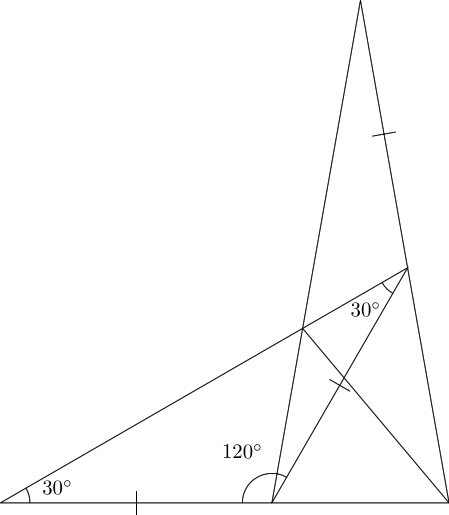Figure 11: Extending the line.

My first thought was that there was a hexagon here somewhere. That proves not to be the case, as although the three marked sides in Figure 11 are the same size, the angles are not all ${120}^{\circ }$. But once one starts thinking about polygons, one starts seeing them everywhere and indeed, there are polygons that can be drawn related to this figure. In particular, the ${20}^{\circ }$ angle at the apex of the outer triangle is the central angle in an $18$–sided polygon. Drawing the triangle adjacent to itself makes two sectors from this polygon. We'll draw them mirrored; the reason for this is that in Figure 3 the line up from the base at ${50}^{\circ }$ "bounces" off the side of the triangle as if in a mirror, so drawing the triangle mirrored next to itself allows us to think of that line continuing straight rather than bouncing. The result is in Figure 12.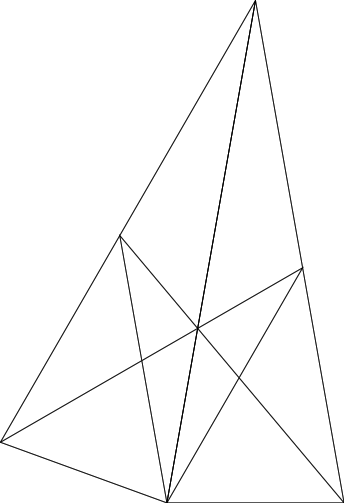Figure 12: Two sectors of an $18$–agon.

Let's simplify that a little and only draw one copy of each line, as in Figure 13. This seems to say that if we draw a line at ${50}^{\circ }$ from one vertex of an $18$–agon and at ${60}^{\circ }$ from the next vertex then they will intercept on a line from the centre to the next vertex after that. The really interesting part is what happens if we continue those lines beyond that intersection point. Both eventually reach other vertices of the $18$–agon: the ${50}^{\circ }$ line reaches the $6$th vertex from its starting point and the ${60}^{\circ }$ line the $7$th from where it began. In Figure 14 we draw the full $18$–agon with just the chords to reveal the structure.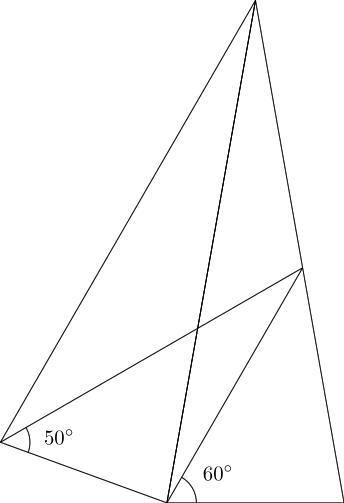Figure 13: Lines from adjacent vertices of an $18$–gon.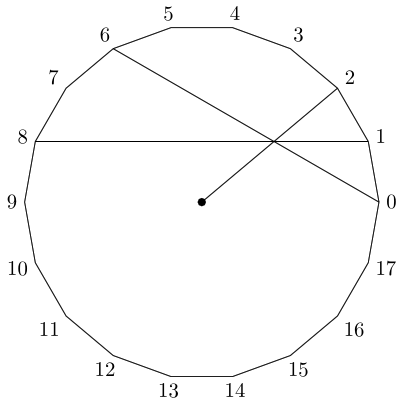Figure 14: Chords in an $18$–gon.

This again looks like quite a coincidence. Experimenting with Geogebra shows that it occurs more often than I would expect. Often enough that it's worth doing a proper search for occurrences to find patterns.

It turns out that there are patterns (the search took me down a considerable side route that was very interesting, but not directly relevant to this problem). The most common pattern occurs when one of the chords makes a ${60}^{\circ }$ angle at the centre, which is exactly what happens here. The other chord has to satisfy properties which boil down to $8=4×2$ and $1=\left(8-6\right)÷2$ (see Figure 14 for the relevance of the numbers $8$, $2$, and $1$).

# 7 Wot No Trig?

The above methods have been interesting to follow but the resulting proofs of the angles are a little backwards. Neither begins with the angles as given but rather start from some other assumption, in both cases about how the inner lines interact, and works backwards to show that all the angles are as stated.

In lieu of anything better, then, the next strategy is to try to work forwards to calculate the missing angle from the given ones. Although the original problem says "No trig allowed", when just solving the problem then artificially limiting oneself is plain dumb. Of course, it can be insightful to then say "How much of that did I really need?", and that is a very common thing for mathematicians to care about. But it really is only afterwards that they do that.

The other reason that I dislike the "No trig allowed" sign is that it can so easily be circumvented, but at the cost of tediousness. Trigonometry is simply about ratios and so every statement using sines and cosines can be reformulated as a statement about similar triangles or other geometric shapes. So if I had a trigonometric argument, I could unpick it to remove the trigonometry by recasting it in more "elementary" terms.4

4I'm young enough to have taken GCSE maths, and it was the system in my school to cover the GCSE syllabus a year early but not to take the exam until the regular time – the intervening year was spent doing an course called "Additional Maths". The GCSE syllabus had no calculus but the Additional Maths one did. I spent some time in one of my GCSE papers wondering if I was allowed to use calculus to answer a particular question.

So let's ignore the "No trig" sign and merrily proceed. As this is meant to be a "How did I do that?" sort of post with details as to how I got there, I'll admit to spending quite some time with the sine rule trying to get one of the unknown angles in a place where I could calculate it. I was trying to avoid the cosine rule (as it introduces more terms), but I did end up briefly trying to put it in. Rather than detail all the blind alleys I went down, I'll just point to Figure 15 as a picture of my working. Somehow, I don't think that that page is going to win any awards for neatness of working or precision of layout.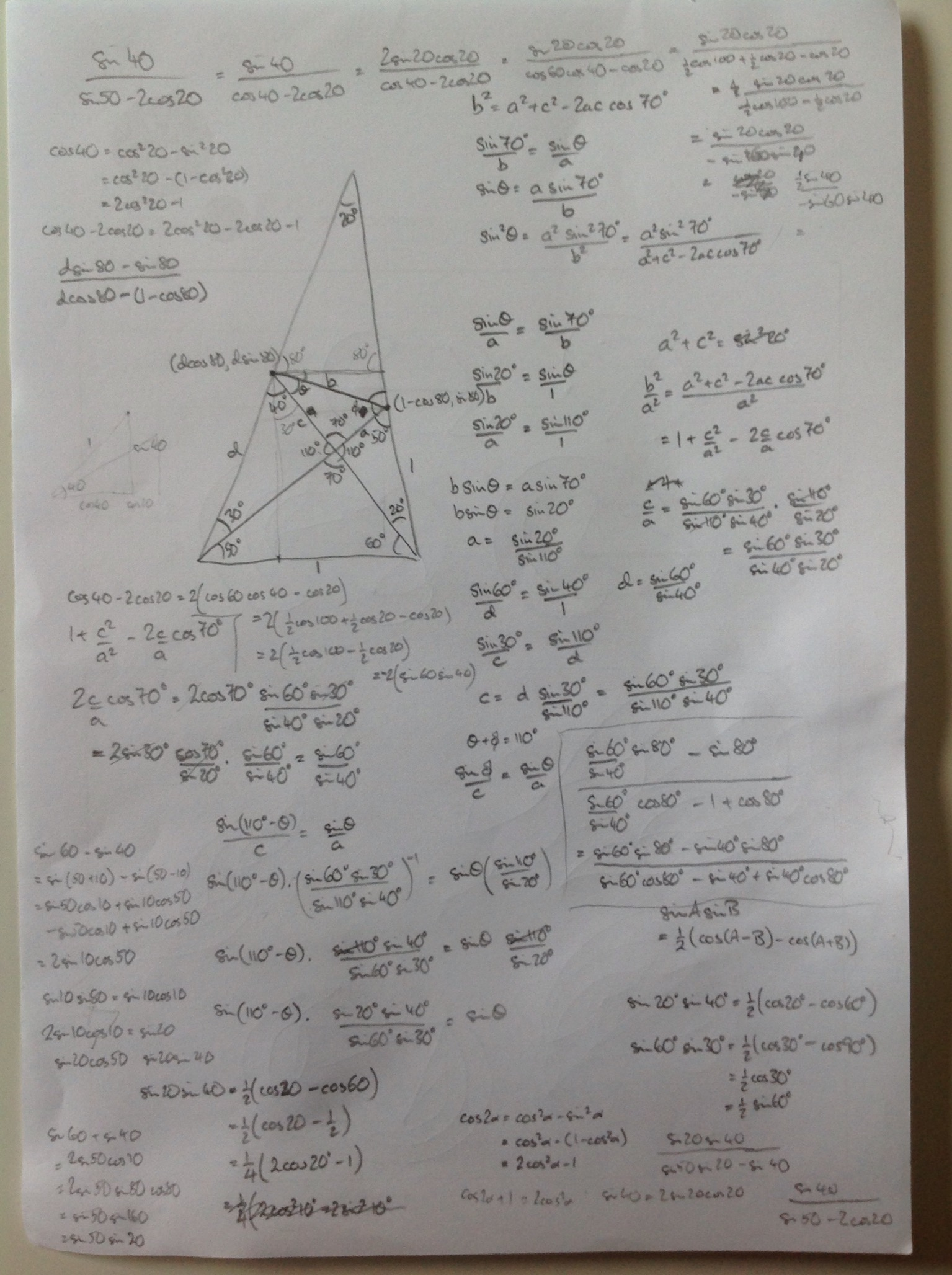Figure 15: My working

Eventually, I decided to work out the gradient of the line which meant finding the coordinates of its endpoints. Again I'll set the base length to $1$ which means that length $AF$ is also $1$, see Figure 16.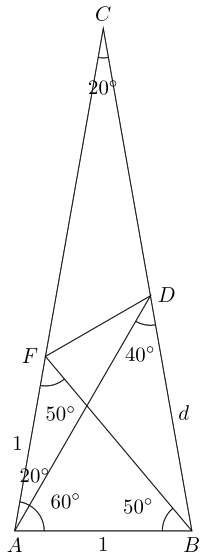Figure 16: Working out the gradient.

We use the sine rule to get length $BD$, labelled $d$ in Figure 16, as:

 $\frac{d}{\mathrm{sin}\left({60}^{\circ }\right)}=\frac{1}{\mathrm{sin}\left({40}^{\circ }\right)}$

With these, we can write down the coordinates of points $F$ and $D$.

 $\begin{array}{rl}F& :\left(\mathrm{cos}\left({80}^{\circ }\right),\mathrm{sin}\left({80}^{\circ }\right)\right)\\ D& :\left(1-d\mathrm{cos}\left({80}^{\circ }\right),d\mathrm{sin}\left({80}^{\circ }\right)\right)\end{array}$

 $m=\frac{d\mathrm{sin}\left({80}^{\circ }\right)-\mathrm{sin}\left({80}^{\circ }\right)}{1-d\mathrm{cos}\left({80}^{\circ }\right)-\mathrm{cos}\left({80}^{\circ }\right)}$

We can simplify this as follows:

 $\begin{array}{rl}m& =\frac{d\mathrm{sin}\left({80}^{\circ }\right)-\mathrm{sin}\left({80}^{\circ }\right)}{1-d\mathrm{cos}\left({80}^{\circ }\right)-\mathrm{cos}\left({80}^{\circ }\right)}\\ & =\frac{\left(d-1\right)\mathrm{sin}\left({80}^{\circ }\right)}{1-\left(d+1\right)\mathrm{cos}\left({80}^{\circ }\right)}\end{array}$

So clearly $d-1$ and $d+1$ would be useful to compute.

 $\begin{array}{rl}d-1& =\frac{\mathrm{sin}\left({60}^{\circ }\right)}{\mathrm{sin}\left({40}^{\circ }\right)}-1\\ & =\frac{\mathrm{sin}\left({60}^{\circ }\right)-\mathrm{sin}\left({40}^{\circ }\right)}{\mathrm{sin}\left({40}^{\circ }\right)}\\ & =\frac{2\mathrm{cos}\left({50}^{\circ }\right)\mathrm{sin}\left({10}^{\circ }\right)}{\mathrm{sin}\left({40}^{\circ }\right)}\\ & =\frac{2\mathrm{sin}\left({40}^{\circ }\right)\mathrm{sin}\left({10}^{\circ }\right)}{\mathrm{sin}\left({40}^{\circ }\right)}\\ & =2\mathrm{sin}\left({10}^{\circ }\right)\end{array}$

Thus the numerator simplifies to:

 $\begin{array}{rl}\left(d-1\right)\mathrm{sin}\left({80}^{\circ }\right)& =2\mathrm{sin}\left({10}^{\circ }\right)\mathrm{sin}\left({80}^{\circ }\right)\\ & =2\mathrm{sin}\left({10}^{\circ }\right)\mathrm{cos}\left({10}^{\circ }\right)\\ & =\mathrm{sin}\left({20}^{\circ }\right)\end{array}$

 $\begin{array}{rl}d+1& =\frac{\mathrm{sin}\left({60}^{\circ }\right)}{\mathrm{sin}\left({40}^{\circ }\right)}+1\\ & =\frac{\mathrm{sin}\left({60}^{\circ }\right)+\mathrm{sin}\left({40}^{\circ }\right)}{\mathrm{sin}\left({40}^{\circ }\right)}\\ & =\frac{2\mathrm{sin}\left({50}^{\circ }\right)\mathrm{cos}\left({10}^{\circ }\right)}{\mathrm{sin}\left({40}^{\circ }\right)}\end{array}$

This isn't quite as nice as the above, but it becomes so when we put in a bit more of the denominator.

 $\begin{array}{rl}\left(d+1\right)\mathrm{cos}\left({80}^{\circ }\right)& =\frac{2\mathrm{sin}\left({50}^{\circ }\right)\mathrm{cos}\left({10}^{\circ }\right)\mathrm{cos}\left({80}^{\circ }\right)}{\mathrm{sin}\left({40}^{\circ }\right)}\\ & =\frac{2\mathrm{sin}\left({50}^{\circ }\right)\mathrm{cos}\left({10}^{\circ }\right)\mathrm{sin}\left({10}^{\circ }\right)}{\mathrm{sin}\left({40}^{\circ }\right)}\\ & =\frac{\mathrm{sin}\left({50}^{\circ }\right)\mathrm{sin}\left({20}^{\circ }\right)}{\mathrm{sin}\left({40}^{\circ }\right)}\\ & =\frac{\mathrm{sin}\left({50}^{\circ }\right)\mathrm{sin}\left({20}^{\circ }\right)}{2\mathrm{sin}\left({20}^{\circ }\right)\mathrm{cos}\left({20}^{\circ }\right)}\\ & =\frac{\mathrm{sin}\left({50}^{\circ }\right)}{2\mathrm{cos}\left({20}^{\circ }\right)}\\ \end{array}$

Thus the denominator in full is:

 $\begin{array}{rl}1-\left(d+1\right)\mathrm{cos}\left({80}^{\circ }\right)& =1-\frac{\mathrm{sin}\left({50}^{\circ }\right)}{2\mathrm{cos}\left({20}^{\circ }\right)}\\ & =\frac{2\mathrm{cos}\left({20}^{\circ }\right)-\mathrm{sin}\left({50}^{\circ }\right)}{2\mathrm{cos}\left({20}^{\circ }\right)}\\ & =\frac{2\mathrm{cos}\left({20}^{\circ }\right)-\mathrm{cos}\left({40}^{\circ }\right)}{2\mathrm{cos}\left({20}^{\circ }\right)}\\ & =\frac{2\mathrm{cos}\left({20}^{\circ }\right)-2\mathrm{cos}\left({60}^{\circ }\right)\mathrm{cos}\left({40}^{\circ }\right)}{2\mathrm{cos}\left({20}^{\circ }\right)}\\ & =\frac{2\mathrm{cos}\left({20}^{\circ }\right)-\left(\mathrm{cos}\left({100}^{\circ }\right)+\mathrm{cos}\left({20}^{\circ }\right)\right)}{2\mathrm{cos}\left({20}^{\circ }\right)}\\ & =\frac{\mathrm{cos}\left({20}^{\circ }\right)-\mathrm{cos}\left({100}^{\circ }\right)}{2\mathrm{cos}\left({20}^{\circ }\right)}\\ & =\frac{2\mathrm{sin}\left({60}^{\circ }\right)\mathrm{sin}\left({40}^{\circ }\right)}{2\mathrm{cos}\left({20}^{\circ }\right)}\\ & =\frac{\mathrm{sin}\left({60}^{\circ }\right)\mathrm{sin}\left({40}^{\circ }\right)}{\mathrm{cos}\left({20}^{\circ }\right)}\\ & =\frac{\mathrm{sin}\left({60}^{\circ }\right)2\mathrm{sin}\left({20}^{\circ }\right)\mathrm{cos}\left({20}^{\circ }\right)}{\mathrm{cos}\left({20}^{\circ }\right)}\\ & =2\mathrm{sin}\left({60}^{\circ }\right)\mathrm{sin}\left({20}^{\circ }\right)\end{array}$

 $\begin{array}{rl}m& =\frac{\mathrm{sin}\left({20}^{\circ }\right)}{2\mathrm{sin}\left({60}^{\circ }\right)\mathrm{sin}\left({20}^{\circ }\right)}\\ & =\frac{1}{2\mathrm{sin}\left({60}^{\circ }\right)}\\ & =\frac{\mathrm{cos}\left({60}^{\circ }\right)}{\mathrm{sin}\left({60}^{\circ }\right)}\\ & =\frac{\mathrm{sin}\left({30}^{\circ }\right)}{\mathrm{cos}\left({30}^{\circ }\right)}\\ & =\mathrm{tan}\left({30}^{\circ }\right)\end{array}$

Thus the line $FD$ is at ${30}^{\circ }$ to the horizontal, and since $CF$ is at ${80}^{\circ }$ to the horizontal, this gives $CFD={50}^{\circ }$.

Incidentally, the one step in the above that is truly from "left field" is throwing in a factor of $2\mathrm{cos}\left({60}^{\circ }\right)\left(=1\right)$. This is where knowing the answer, and having explored the problem with other strategies, helps: we have to get ${30}^{\circ }$ or ${60}^{\circ }$ in there somewhere so as it wasn't there at the outset we have to find some place to put it in.

It's also another example of a habit of mathematicians of doing nothing in an interesting way. That is, we multiply something by $1$ – which accomplishes nothing – but in an interesting way – by writing $1=2\mathrm{cos}\left({60}^{\circ }\right)$.

# 8 Introducing Triangles

The previous section has an intriguing implication. It's not hard to work out from the above calculation that the line $FD$ has length $2\mathrm{sin}\left({20}^{\circ }\right)$. A formula of the type $2\mathrm{sin}\left(\theta /2\right)$ occurs when working out the base length of an isosceles triangle with apex angle $\theta$. So this suggests that the line $FD$ is the base of an isosceles triangle with apex angle ${40}^{\circ }$ and other side length $1$. If the apex angle is ${40}^{\circ }$ then the base angle is ${70}^{\circ }$. The angle $FDB$ is ${70}^{\circ }$, so that means that one of the sides of the isosceles triangle lies along $DB$, though $DB$ is too long itself. So let's mark a new point at the apex of this (upside down) isosceles triangle. This is in Figure 17.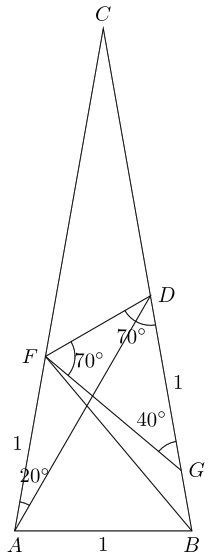Figure 17: The new isosceles triangle.

As this is meant to be a write-up of a process rather than a conclusion, I should admit here to spending a bit of time staring at a parallelogram before realising that the right thing to do was something else. If one draws a line through $F$ parallel to $DG$ and marks where that intersects with the line $AB$, say $H$, then the resulting shape $FHGD$ is a parallelogram. I thought it might be possible to work with the new line $HG$ instead of $FD$, but in the end found an alternative route which didn't involve this new point.

In Figure 17, as well as the angles in the triangle $DFG$ I've marked angle $DAF$. The reason for doing this is that it is half of angle $DGF$. As the triangle $DFG$ is isosceles, this means that a circle centred at $G$ with radius $1$ will pass through both $D$ and $F$ making $DF$ a chord of this circle. That angle $DAF$ is half of $DGF$ and they are on the same side of $DF$ means that $A$ is also on this circle, whence in particular the length of $AG$ is $1$.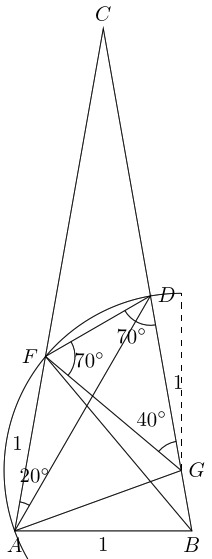Figure 18: Introducing a circle.

And now all angles are calculable. As $AG$ has length $1$, $AGF$ is an equilateral triangle. Also $ABG$ is an isosceles triangle, meaning that angle $BAG$ is ${20}^{\circ }$ (since we know that $GBA$ is ${80}^{\circ }$).

If you'll pardon the pun, the point $G$ seems fairly central to the whole problem.

# 9 Solving the Puzzle

In fact, the point $G$ finally provides us with what we want. In all the above, although we've found ways to calculate one, and hence all, of the missing angles, our routes have been backwards. We've found some construction that gives us a line across the triangle that we can compute the angle for and then shown that that line was our original one. With none of the constructions have we been able to start from the original diagram and show that the angle is what it is by "simple" means.

But the addition of the point $G$ provides this. So first we'd better "find" it in our original diagram5.

5There's a Calvin and Hobbes cartoon where Calvin uses a treasure map to find some treasure. When Hobbes expresses admiration for the fact that the map worked, Calvin lets on that he buried the treasure in the first place. That is exactly what we're doing here: we're going to "dig up" the point $G$, knowing exactly where it is all along.

A common theme in all of this has been the importance of the angle ${60}^{\circ }$, but it hasn't always been the ${60}^{\circ }$ in the original diagram that's been the key. Our equilateral triangle gives us another ${60}^{\circ }$ (in fact three of them), so let's use this to "find" $G$. We'll choose the point $G$ so that $AG$ has length $1$, as in Figure 19.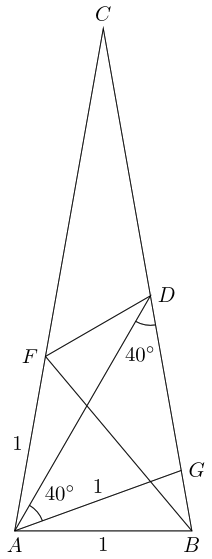Figure 19: Finding $G$.

This means that triangle $ABG$ is isosceles and so angle $GAB$ is ${20}^{\circ }$. Since $BAD$ is ${60}^{\circ }$, this leaves $GAD$ as ${40}^{\circ }$. We already know that $ADB$ is ${40}^{\circ }$ so $AGD$ is an isosceles triangle with $G$ at the apex. Hence $DG$ has length $1$.

Futhermore, angle $GAF$ is ${60}^{\circ }$ and triangle $GAF$ is isosceles, whence equilateral. So the line $GF$ also has length $1$. Drawing a circle centred on $G$ therefore passes through $D$, $F$, and $A$.

At this point, we have reconstructed Figure 18 without calculating any of the angles inside triangle $DFG$. As we do know angle $DAF$, we can use the fact that $DF$ is a chord of this circle to see that angle $DGF$ is ${40}^{\circ }$ and proceed from there to calculate all the missing angles.

This is now a direct calculation of the missing angles using only knowledge of isosceles triangles and that the angle subtended6 at the centre of a circle is twice that at the circumference. I deem these facts sufficiently "basic" for this to qualify as a solution to the original puzzle.

6I've never come across this word in any other context.

# 10 Generalising the Puzzle

Now that we have an acceptable solution, we can see where we went wrong with all our attempts to generalise the puzzle at the start. It is clear from the solution that the key is all the lines of length $1$, and all of those emanate either from $A$ or from $G$. So the real starting place of this puzzle is the points $A$ and $G$ and two circles as in Figure 20.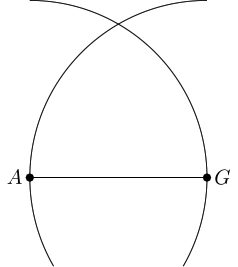Figure 20: The starting circles.

From this, we create the obvious equilateral triangle which is the kernel of the puzzle. We also need one more point which we'll choose to be the point $B$. This must lie on the circle centred at $A$ and be a little further round than $G$, as in Figure 21.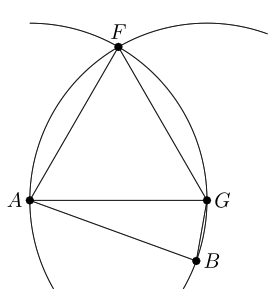Figure 21: The initial configuration.

To create the puzzle, we extend the lines $AF$ and $BG$ until they meet. The line $BG$ will intersect the circle centred at $G$ and we mark this point $D$, as in Figure 22.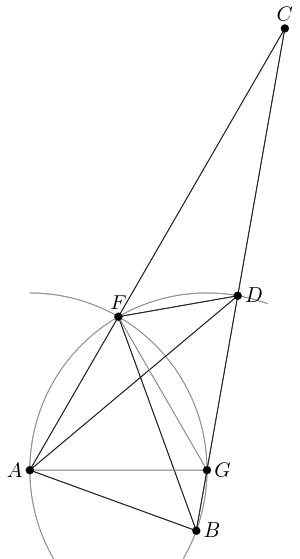Figure 22: Constructing the general puzzle.

The one choice to make in this is the position of $B$. That is determined by the angle $BAG$, so let us label that $\psi$. From that one angle we can calculate all of the others.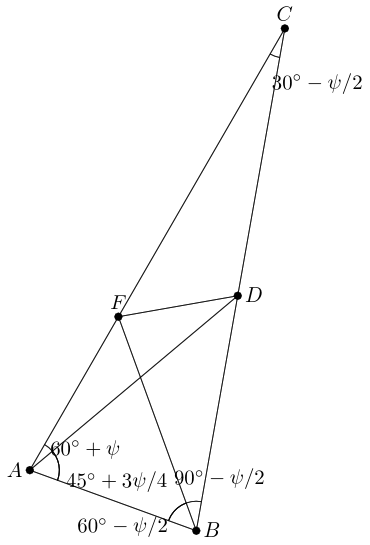Figure 23: The general puzzle.

Setting $\psi ={20}^{\circ }$ yields the original puzzle. Also, insisting that the outer triangle be isosceles forces the original puzzle since it implies that ${90}^{\circ }-\psi /2={60}^{\circ }+\psi$ from which we get $\psi ={20}^{\circ }$.

Alternative "nice" puzzles would be with right-angled triangles. There are two for this, one with $\psi ={0}^{\circ }$ which is in Figure 24 and one with $\psi ={30}^{\circ }$ which is in Figure 25. The solutions for these are ${x}^{\circ }={45}^{\circ }$ and ${x}^{\circ }={52.5}^{\circ }$ respectively.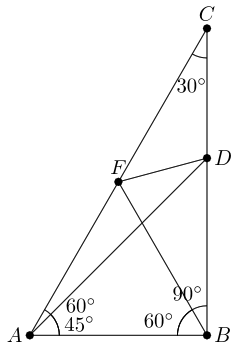Figure 24: One right-angled version of the puzzle.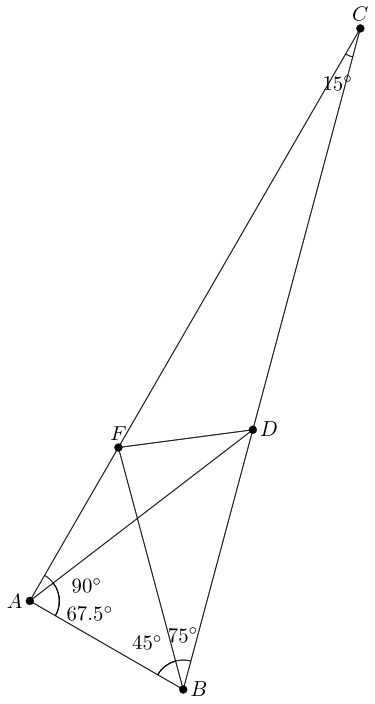Figure 25: Another right-angled version of the puzzle.

The range for $\psi$ is from ${0}^{\circ }$ to ${60}^{\circ }$. At $\psi ={60}^{\circ }$, the lines $AF$ and $BG$ are parallel and so the construction fails.

It is perhaps worth noting that since the range of values for the solution, ${x}^{\circ }=DFC$, is between ${45}^{\circ }$ and ${60}^{\circ }$ (with ${60}^{\circ }$ being not possible), then ${x}^{\circ }={50}^{\circ }$ is the "nicest" solution as the only multiple of $10$ that is possible.

# 11 And Finally …

Having finally "solved" the puzzle, it's time to look up the puzzle. It has a page on Wikipedia. My solution matches the official one, and the generalisation given there is closely related to the polygonal approach that I found7. There's no mention of the superposition approach, so I'm claiming that one!

7which, via searching, led me to the article The Number of Intersection Points made by the Diagonals of a Regular Polygon by Björn Poonen and Michael Rubinstein. This is now on my list of articles to read.

In the end, the point was to solve this problem as a mathematician and not as a puzzler. By doing so, I was able to use the puzzle as a launching point for an exploration of a region of mathematics that I might not have encountered otherwise. I spent quite some time in that exploration, and enjoyed it thoroughly.

So when next you are faced with a mathematical puzzle, look at it as a mathematician, not as a puzzler. Here's my guide to keep you on a mathematical track:

1. Ignore any artificial constraints.

The problem is yours to use as you like, not someone else's to impose on you.

2. Get to the answer by any means possible and then work backwards as well as forward.

It's always easiest to travel if you can see both where you started and where you want to end up. It's also easier to explore off the beaten track if you have beacons to find your way home again.

3. Follow up all the "What if …?" thoughts that occur to you.

4. Follow up all the things that provoke the reation "Whoa, I didn't expect that!"

5. Above all, when you find a more elegant path or neater argument, don't feel bad for not spotting it before. If you hadn't put in all the work beforehand you wouldn't appreciate just how elegant the new path actually is.